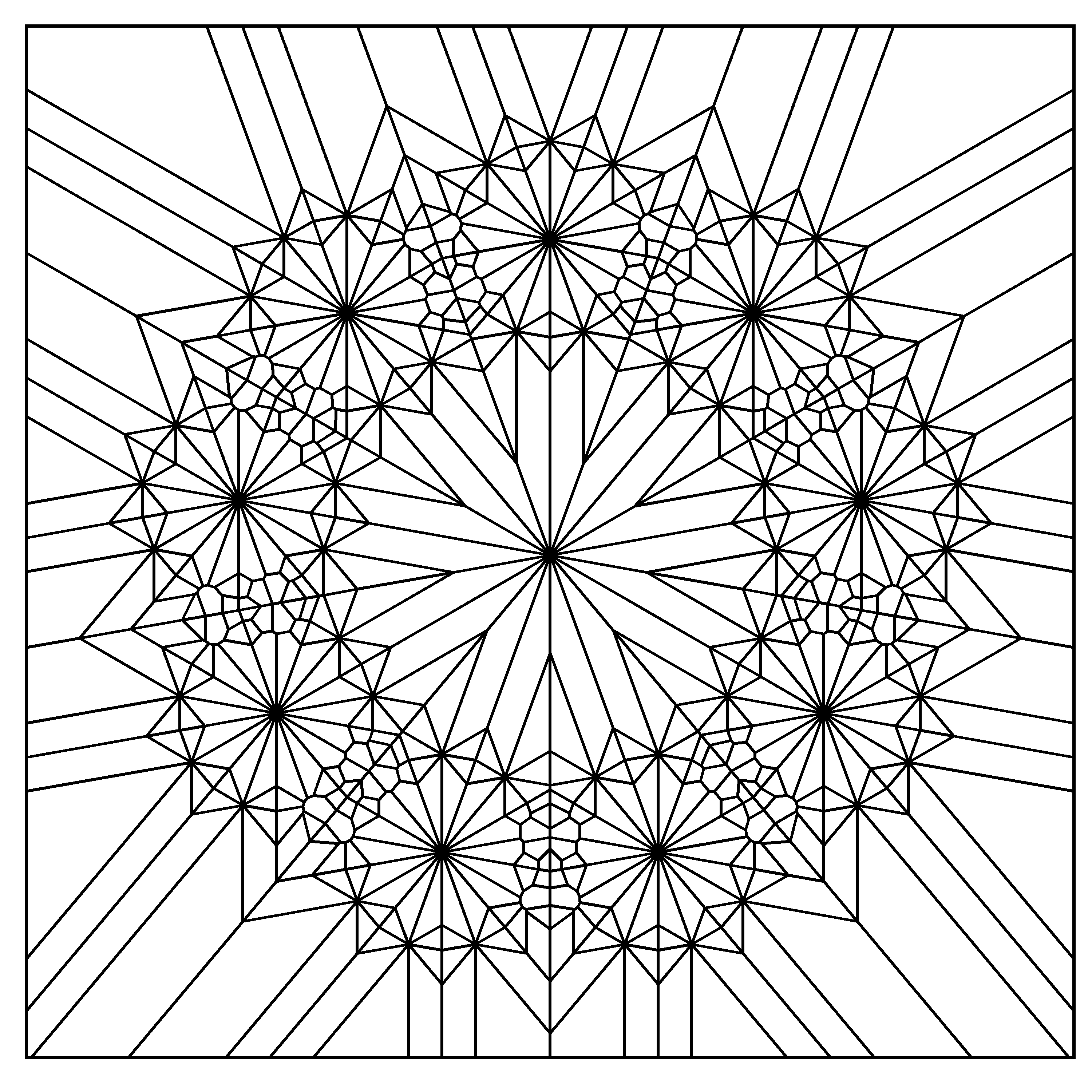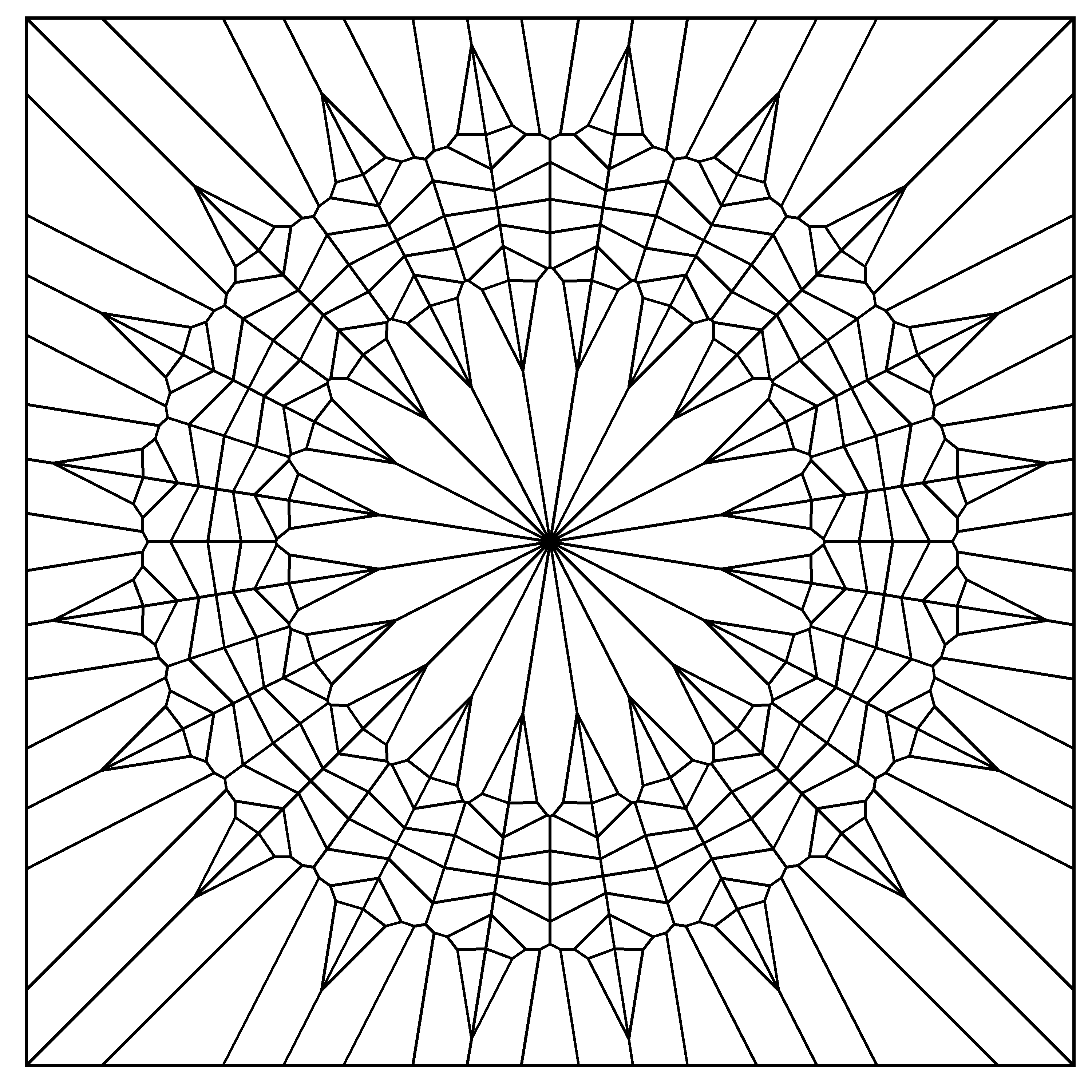Want to share your content on R-bloggers? click here if you have a blog, or here if you don't.

Mathematics is a place where you can do things which you can’t do in the real world (Marcus Du Sautoy, mathematician)

From time to time I have a look to some of my previous posts: it’s like seeing them through another’s eyes. One of my first posts was this one, where I draw fractals using the Multiple Reduction Copy Machine (MRCM) algorithm. That time I was not clever enough to write an efficient code able generate deep fractals. Now I am pretty sure I could do it using `ggplot` and I started to do it when I come across with the idea of mixing this kind of fractal patterns with Voronoi tessellations, that I have explored in some of my previous posts, like this one. Mixing both techniques, the mandalas appeared.

I will not explain in depth the mathematics behind this patterns. I will just give a brief explanation:

• I start obtaining `n` equidistant points in a unit circle centered in `(0,0)`
• I repeat the process with all these points, obtaining again `n` points around each of them; the radius is scaled by a factor
• I discard the previous (parent) `n` points

I repeat these steps iteratively. If I start with n points and iterate k times, at the end I obtain nk points. After that, I calculate the Voronoi tesselation of them, which I represent with `ggplot`.

This is an example:Some others:You can find the code here. Enjoy it.